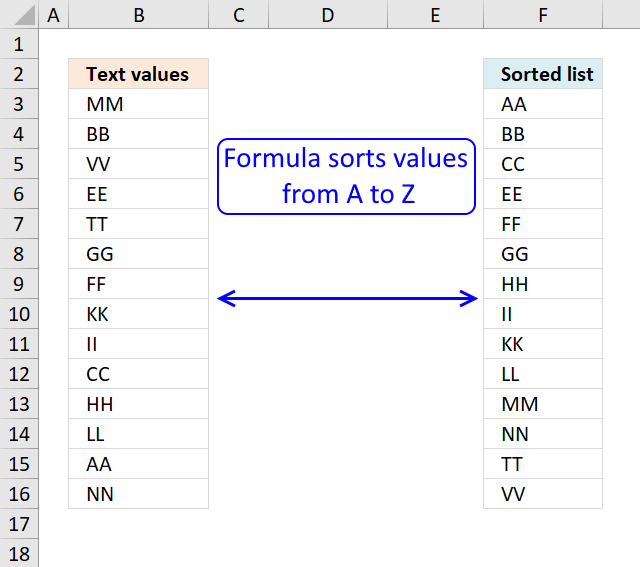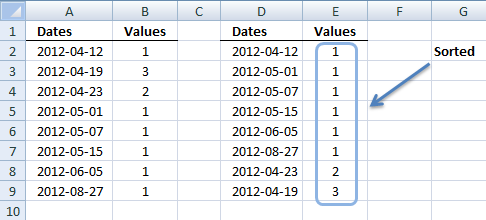Author: Oscar Cronquist Article last updated on October 31, 2018I am inspired once again by the article Sorting Text in Excel using Formulas at Pointy haired Dilbert. In Chandoo´s article he sorts text with a "helper" column. My goal with this article is to show you how to sort text cells alphabetically without a helper column.

Here is an example, cell range \$A\$2:\$A\$15 contains text values.

Here is how to automatically sort text cells without any user interaction in cell B2:

Array formula in cell B2:

=INDEX(\$A\$2:\$A\$15, MATCH(SMALL(COUNTIF(\$A\$2:\$A\$15, "<"&\$A\$2:\$A\$15), ROW(1:1)), COUNTIF(\$A\$2:\$A\$15, "<"&\$A\$2:\$A\$15), 0))

Sort both numerical and text values with blank cells:

This article demonstrates how to filter a unique distinct list sorted from A to Z:

Extract a unique distinct list sorted from A to Z

The array formula in cell D3 extracts unique distinct values sorted A to Z, from column B to column D. […]

The following post shows you how to sort and filter duplicate values alphabetically:

Extract a list of alphabetically sorted duplicates from a column

The following array formula extracts duplicate values sorted from A to Z from cell range B3:B21. Excel array formula in […]

Learn how to sort a multi-column cell range alphabetically and display the values in a single column:

Sort a range from A to Z [Array formula]

Question: How do I sort a range alphabetically using excel array formula? Answer: Cell range \$B\$2:\$E\$5 contains text values in random […]

Sort a data set using an array formula:

How to sort a data set using three different approaches, built-in tools, array formulas, and VBA

I will in this article demonstrate three different techniques to sort a data set in Excel. The first method sorts […]

Tip! You can sort a data set in any way you like it, if you convert it to an excel defined table: Excel table - Sort data

### How to create an array formula

1. Copy (Ctrl + c) and paste (Ctrl + v) array formula into formula bar.
2. Press and hold Ctrl + Shift.
3. Press Enter once.
4. Release all keys.

Copy cell B2 and paste it down as far as needed.

Recommended article

A beginners guide to Excel array formulas

Array formulas allows you to do advanced calculations not possible with regular formulas.

### Explaining array formula in cell B2

=INDEX(\$A\$2:\$A\$15, MATCH(SMALL(COUNTIF(\$A\$2:\$A\$15, "<"&\$A\$2:\$A\$15), ROW(1:1)), COUNTIF(\$A\$2:\$A\$15, "<"&\$A\$2:\$A\$15), 0))

Step 1 - Count "smaller" values

COUNTIF(range,criteria) counts the number of cells within a range that meet the given condition

=INDEX(\$A\$2:\$A\$15, MATCH(SMALL(COUNTIF(\$A\$2:\$A\$15, "<"&\$A\$2:\$A\$15), ROW(1:1)), COUNTIF(\$A\$2:\$A\$15, "<"&\$A\$2:\$A\$15), 0))

COUNTIF(\$A\$2:\$A\$15, "<"&\$A\$2:\$A\$15)

becomes

COUNTIF({MM,BB,VV,EE,TT,GG,FF,KK,KK,II,CC,HH,LL,AA,NN}, "<"&MM,"<"&BB,"<"&VV,"<"&EE,"<"&TT,"<"&GG,"<"&FF,"<"&KK,"<"&II,"<"&CC,"<"&HH,"<"&LL,"<"&AA,"<"&NN)

The first value in this array formula is "MM". Let´s see what happens when COUNTIF calculates how many values is small than "MM".

COUNTIF({MM,BB,VV,EE,TT,GG,FF,KK,II,CC,HH,LL,AA,NN}, "<"&"MM"

becomes

COUNTIF({MM"<"&"MM",BB"<"&"MM",VV"<"&"MM",EE"<"&"MM",TT"<"&"MM",GG"<"&"MM",FF"<"&"MM",KK"<"&"MM",II"<"&"MM",CC"<"&"MM",HH"<"&"MM",LL"<"&"MM",AA"<"&"MM",NN"<"&"MM"}

MM<MM is FALSE and BB<MM is TRUE and so on.. The array becomes:

COUNTIF({FALSE, TRUE, FALSE, TRUE, FALSE, TRUE, TRUE, TRUE, TRUE, TRUE, TRUE, TRUE, TRUE, FALSE}

becomes

COUNTIF({0, 1, 0, 1,0, 1 , 1 ,1 ,1 ,1 ,1 ,1 ,1 ,1 ,1 ,1 ,0} and the total is 10 which is the first number in the returning array. 0+1+0+1+0+1+1+1+1+1+1+1+1+1+1+1+0 = 10

Next is COUNTIF({MM,BB,VV,EE,TT,GG,FF,KK,II,CC,HH,LL,AA,NN}, "<"&"BB")

becomes

COUNTIF({MM"<"&"BB",BB"<"&"BB",VV"<"&"BB",EE"<"&"BB",TT"<"&"BB",GG"<"&"BB",FF"<"&"BB",KK"<"&"BB",II"<"&"BB",CC"<"&"BB",HH"<"&"BB",LL"<"&"BB",AA"<"&"BB",NN"<"&"BB"})

becomes

COUNTIF({FALSE, FALSE, FALSE, FALSE, FALSE, FALSE, FALSE, FALSE, FALSE, FALSE, FALSE,FALSE , TRUE, FALSE})

becomes

COUNTIF({0, 0, 0, 0,0, 0, 0,0,0,0,0,0,0,0,0,1,0}) and the total is 1 which is the second number in the returning array.

and so on...

COUNTIF(\$A\$2:\$A\$15, "<"&\$A\$2:\$A\$15) returns this array:

{10, 1,13,3,12,5,4,8,7,2,6,9,0,11}

Recommended article

How to use the COUNTIF function

Counts the number of cells that meet a specific condition.

Step 2 - Return the k-th smallest number in array

SMALL(array,k) returns the k-th smallest row number in this data set.

SMALL({10, 1,13,3,12,5,4,8,7,2,6,9,0,11}, ROW(1:1)) returns the smallest number in this data set, 0 (zero).

Recommended article

How to use the SMALL function

The SMALL function lets you extract a number in a cell range based on how small it is compared to the other numbers in the group.

Step 3 - Find position in array

MATCH(lookup_value;lookup_array; [match_type]) returns the relative position of an item in an array that matches a specified value

MATCH(SMALL(COUNTIF(\$A\$2:\$A\$15, "<"&\$A\$2:\$A\$15), ROW(1:1)), COUNTIF(\$A\$2:\$A\$15, "<"&\$A\$2:\$A\$15), 0)

becomes

MATCH(0, {10, 1,13,3,12,5,4,8,7,2,6,9,0,11}, 0) and returns 13.

Recommended article

How to use the MATCH function

Identify the position of a value in an array.

Step 4 - Return value

INDEX(array,row_num,[column_num]) returns a value or reference of the cell at the intersection of a particular row and column, in a given range.

=INDEX(\$A\$2:\$A\$15, MATCH(SMALL(COUNTIF(\$A\$2:\$A\$15, "<"&\$A\$2:\$A\$15), ROW(1:1)), COUNTIF(\$A\$2:\$A\$15, "<"&\$A\$2:\$A\$15), 0))

becomes

=INDEX(\$A\$2:\$A\$15, 13) and returns AA in cell B2.

Recommended article

How to use the INDEX function

Gets a value in a specific cell range based on a row and column number.

### Get excel sample file for this tutorial

Sorting-text-cells-using-array-formulas.xls
(Excel 97-2003 Workbook *.xls)

Recommended posts

Sort text cells alphabetically from two columns

Table of Contents Sort text from two columns combined (array formula) How to create an array formula How to copy […]

Populate drop down list with unique distinct values sorted from A to Z

Question: How do I create a drop-down list with unique distinct alphabetically sorted values? Table of contents Sort values using […]

## Two columns sorting by the second columnArray Formula in cell E2:

=INDEX(\$B\$2:\$B\$9, MATCH(SMALL(COUNTIF(\$B\$2:\$B\$9, "<"&\$B\$2:\$B\$9), ROW(1:1)), COUNTIF(\$B\$2:\$B\$9, "<"&\$B\$2:\$B\$9), 0))

Array Formula in cell D2:

=INDEX(\$A\$2:\$A\$9, SMALL(IF(E2=\$B\$2:\$B\$9, MATCH(ROW(\$B\$2:\$B\$9), ROW(\$B\$2:\$B\$9))), SUM(--(E2=\$E\$2:E2))))

### Get excel *.xlsx

Two columns sorting by the second column.xlsx